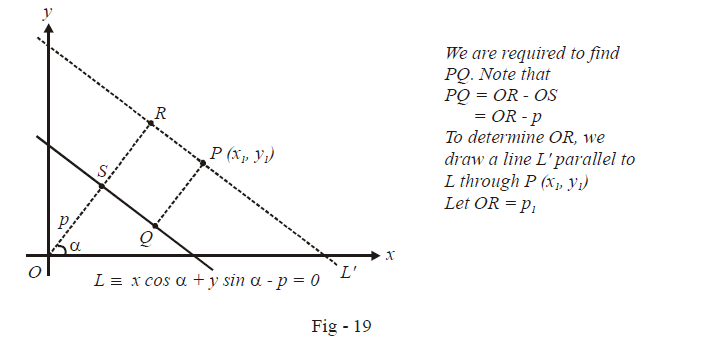# Length of Perpendicular from a Point to a Line

$$\textbf{Art 7 : } \qquad\boxed{{\text{Length of perpendicular}}}$$

Suppose that we are given the equation of a line L and we are required to find the length of the perpendicular dropped from an arbitrary point  $$P\left( {{x_1},{y_1}} \right)\,\,{\text{on}}\,\,L.$$

Suppose that the equation of L is in normal form, i.e,$$L \equiv x\cos \alpha + y\sin \alpha = p.$$Based on the discussion in the figure above, the equation of the line $${L^{\,{\mathbf{'}}}}\,\,{\text{is}}\,\,x\cos \alpha + y\sin \alpha - {p_1} = 0.$$ Since L1 passes through P, the co-ordinates of P must satisfy the equation of L1. Thus,

${x_1}\cos \alpha + {y_1}\sin \alpha - {p_1} = 0$

Thus, we get $${p_1}$$ as $$\left( {{x_1}\cos \alpha + {y_1}\sin \alpha } \right).$$ The length of perpendicular PQ is now simply $$\left| {{p_1} - p} \right| = \left| {{x_1}\cos \alpha + {y_1}\sin \alpha - p} \right|$$. (Modulus sign is used since PQ is a length so it must be positive).

$\boxed{PQ = \left| {{x_1}\cos \alpha + {y_1}\sin \alpha - p} \right|}\,\,\,\,:\,\,\,\,{\mathbf{Length}}{\text{ }}{\mathbf{of}}{\text{ }}{\mathbf{perpendicular}}$

Let us now assume the case where L is given in the general form, i.e.$$L \equiv ax + by + c = 0.$$

We can easily adjust the equation of L so that c is negative. We do this so that we can convert L into the normal form:

\begin{align}& \,\,\,\,\,\,\,\,\,\,\,\,\,\,\,\,\qquad ax + by + c = 0\qquad c < {\text{ }}0 \\&\qquad \Rightarrow \quad ax + by = - c \\&\qquad\Rightarrow \quad \left( {\frac{a}{{\sqrt {{a^2} + {b^2}} }}} \right)x + \left( {\frac{b}{{\sqrt {{a^2} + {b^2}} }}} \right)y = \left( {\frac{{ - c}}{{\sqrt {{a^2} + {b^2}} }}} \right) \\&\qquad \Rightarrow \quad x\cos \alpha + y\sin \alpha = p\qquad \qquad \qquad \qquad \qquad \qquad \qquad ...{\text{ }}\left( 1 \right) \\& {\text{where}}\;\;\;\cos \alpha = \frac{a}{{\sqrt {{a^2} + {b^2}} }},\,\,\sin \alpha = \frac{b}{{\sqrt {{a^2} + {b^2}} }}\,\,{\text{and}}\,\,p = \frac{{ - c}}{{\sqrt {{a^2} + {b^2}} }} \end{align}

The equation in (1) is in the normal form; we can now use the result obtained in the preceding discussion to obtain the length of the perpendicular PQ:

\begin{align}& PQ = \left| {{x_1}\cos \alpha + {y_1}\sin \alpha - p} \right|\,\,\,\,\left\{ \begin{gathered} {\text{Modulus sign is}} \\ {\text{used since }}PQ \\ {\text{must be + ve}} \end{gathered} \right\} \\ & \qquad = \left| {\frac{{a{x_1}}}{{\sqrt {{a^2} + {b^2}} }} + \frac{{b{y_1}}}{{\sqrt {{a^2} + {b^2}} }} + \frac{c}{{\sqrt {{a^2} + {b^2}} }}} \right| \\& \boxed{PQ = \frac{{\left| {a{x_1} + b{y_1} + c} \right|}}{{\sqrt {{a^2} + {b^2}} }}}\qquad :\qquad {\mathbf{Length}}{\text{ }}{\mathbf{of}}{\text{ }}{\mathbf{perpendicular}} \end{align}